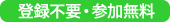### 第23回統計地震学セミナー

2011年12月12日（月）/ 12 December 2011 (Monday)16:00-17:00

Room D312A @ Institute of Statistical Mathematics

Infinitesimal analysis of set-valued functions and applications to spacial statistics and image analysis

(Speaker)
Prof. Khmaladze, Estate V.
（School of Mathematics, Statistics and Operations Research,
Victoria University of Wellington, New Zealand.・Professor）

The problem under consideration started with a study of spacial change-point problem, or change-set problem as we called it: suppose there is a set $K$, such that our observations inside this set behave differently then anywhere outside it. Given $n$ observations, how can we test that a given $K$ is the correct one against its small perturbation $K(\varepsilon)$ as an alternative?

In huge amount of publications on the change-set problem, almost all devoted to the estimation of the change-set, we could not find such an object as an "alternative set" $K(\varepsilon)$. One reason for this porbably is that it is not easy to realize how to describe small changes in sets. We developed an appropriate notion of the derivative of set-valued function in (Khmaladze, 2007) and used it to build a version of contiguity theory in (Einmahl, Khmaladze, 2011) for statistical problems where the parameter of interest is a set.

One single result here is that if $\Phi_n$ is a point process with increasing intenstiy $n$, and the symmetric difference $K(\varepsilon)\Delta K$ shrinks and "vanishes" as $\varepsilon \to 0$, then the sequence $\Phi_n(K(\varepsilon)\Delta K)$ lives in the limit on the derivative set $dK(\varepsilon)/d\varepsilon$:

$$\Phi_n( K(\varepsilon)\Delta K) \to \Psi (dK(\varepsilon)/d\varepsilon, {\rm as} n\to\infty, \varepsilon\to 0.$$

In the talk we present the main framework of this approach. We hope that some discussions would lead to further applications.